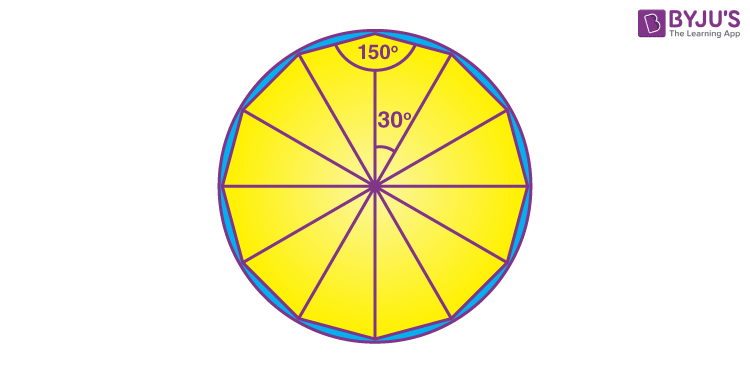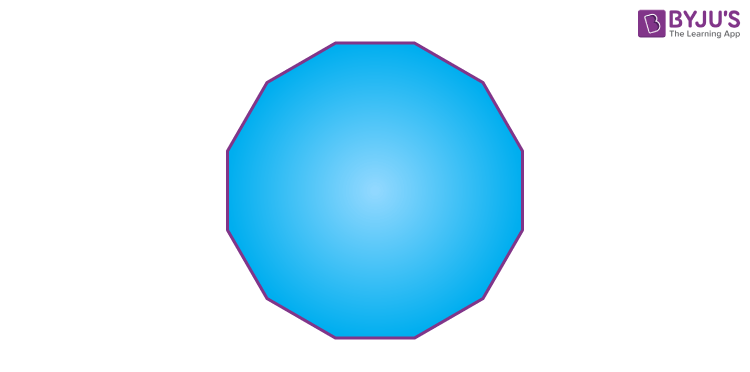# Dodecagon

Dodecagon is one of the types of polygons that has 12 sides, 12 vertices and 12 angles. Similar to other polygons, a dodecagon is also a two-dimensional plane figure. A regular dodecagon polygon has 12 equal sides and has 12 equal measures of angles. Irregular dodecagons have unequal sides and angles. Also, a dodecagon can be a convex polygon or concave polygon. The sum of all the interior angles of the dodecagon is equal to 1800°.

The word dodecagon is derived from Greek words, ‘dodeka‘ which means ‘twelve’ and ‘gon‘ means ‘sides’. Here, we will discuss the properties, sides, angles, area and perimeter of the twelve-sided polygon (dodecagon).

## What is a Dodecagon?

A dodecagon is a closed figure that has 12 sides and 12 angles. It has 12 vertices, each of which is connected to two sides.  See the below figure which is an example of a dodecagon.Depending on the sides and angles a dodecagon can be a regular or irregular polygon and a convex or concave polygon. Let us now discuss the types of a dodecagon.

## Types of Dodecagon

There are basically four types of a dodecagon, they are:

• Regular dodecagon
• Irregular dodecagon
• Convex dodecagon
• Concave dodecagon

Let us discuss them all one by one.

### Regular dodecagon

A regular dodecagon has all its 12 sides equal in length and all the angles have equal measures. All the 12 vertices are equidistant from the center of the dodecagon. For a regular dodecagon each of its interior angle measure 150°.

A regular dodecagon is a symmetrical polygon.

### Irregular dodecagon

An irregular dodecagon does not have all its 12 sides equal in length. Also, the measure of 12 angles of an irregular dodecagon, may vary from each other. It is an unsymmetrical polygon.

### Convex dodecagon

A usual dodecagon that has all its vertices pointed outside the center is a convex polygon. No line segments between the vertices go inside the convex dodecagon. All the interior angles will be less than 180° here.### Concave dodecagon

If any one of the interior angles of a dodecagon is greater than 180 degrees, then it is called a concave dodecagon. We may also say if any of the vertices are pointed towards the center or inwards, then it is a concave dodecagon.## Properties of a Dodecagon

• A dodecagon has 12 sides, 12 vertices and 12 angles
• Each interior angle is equal to 150° and each exterior angle is equal to 30°.
• Interior angles: The sum of interior angles of a twelve-sided polygon (dodecagon) is = (12 – 2) x 180° = 1800°.
• Exterior angles: The sum of the exterior angles of a twelve-sided polygon (dodecagon) is 360°.
• Diagonals in dodecagon: The number of all possible diagonals in a twelve-sided polygon is given by the formula:

Total diagonals = n(n – 3)/2 = 12(12 – 3)/2 = 6 × 9 = 54

• Triangles in dodecagon: The number of triangles formed by the diagonals from each vertex of a twelve-sided polygon is, n – 2 = 12 – 2 = 10.

## Area of Dodecagon

The total region covered inside the boundary of the Dodecagon is called the area of a Dodecagon. It is given by:

Area = ½ × perimeter × apothem

The formula for area of a regular twelve-sided regular polygon (dodecagon) of side length d is given by:

 Area = 3(2+√3)d2 ≈ 11.19615242 d2

The area calculated in terms of circumradius R of the circumscribed circle is;

 Area = 3R2

## Perimeter of Dodecagon

The perimeter is the total length of the boundaries of a twelve-sided polygon. The perimeter formula of dodecagon in terms of circumradius R is given by;

 Perimeter = 12R√(2-√3) ≈ 6.2116570 R

## Facts on Dodecagon

 Number of sides 12 Number vertices 12 Number of angles 12 Interior angle 150° Exterior angle 30° Area ½ × perimeter × apothem or 3(2+√3)d2 Perimeter 12 × side Sum of interior angles 1800°

## Solved Examples on Dodecagon

Q. 1: Calculate the area of the dodecagon with side length d = 10 cm.

Solution:

Number of sides = 12

Area of 12 sided polygon = 3(2+√3)d2

= 3(2+√3) x 102

= 11.19615242 x 100

Area ≈ 1119.615242 cm2

Q.2: Calculate the perimeter of a twelve-sided polygon, which is circumscribed by a radius of 5cm.

Solution: Given, radius of circumcircle = 5cm.

The formula for perimeter of dodecagon is;

P = 12R√(2-√3)

= 12 x 5 x √(2-√3)

P ≈ 31.058285 cm

Q.3: What is the perimeter of a regular dodecagon, that has a side length equal to 3.5 cm?

Solution: Given, the side-length = 3.5 cm

Perimeter = 12 x side = 12 x 3.5 = 42 cm

### Practice Questions on Dodecagon

1. Find the area of a dodecagon, whose each of its sides is equal to 5 cm.
2. Find the perimeter of a dodecagon, whose each of its side is equal to 7cm.

## Frequently Asked Questions on Dodecagon

Q1

### What is a dodecagon?

A dodecagon is a closed figure, that has 12 sides, 12 angles and 12 vertices.
Q2

### What is the interior angle of dodecagon?

If a dodecagon is a regular polygon, the measure of each angle is equal to 150 degrees.
Q3

### What is the sum of all the interior angles of dodecagon?

The sum of all the interior angles of dodecagon is 1800 degrees.
Q4

### What is the sum of all exterior angles of dodecagon?

The sum of all the exterior angles of dodecagon is 360 degrees.
Q5

### How many triangles are formed in a dodecagon?

There is a total of 10 triangles formed in a dodecagon.
Q6

### What is the number of diagonals formed in dodecagon?

There are a total of 54 diagonals formed in a dodecagon.
Q7

### What are the types of dodecagon?

There are four types of dodecagon, they are regular dodecagon, irregular dodecagon, convex dodecagon and concave dodecagon.
Q8

### What is the area of dodecagon?

The area of the dodecagon is ½ × perimeter × apothem or 3(2+√3)d2.